Top 5 Things to know about using arrays to model multiplication & division:
1. An array is an arrangement of things organized into equal rows and equal columns, which creates a rectangular shape. The rows represent the number of groups and the columns represent the number in each group.Tools that are good for modeling arrays include: counters, square tiles, graph paper, and quick pictures. Using arrays to model division or multiplication problems, we want to move from concrete manipulatives, to quick pictures, to abstract thinking using expressions or equations to describe the arrays.

This is a concrete model of an array. This could be the model of a division problem involving 15 ÷ 5 or 15 ÷ 3. This could also be the model for the multiplication problem 5 x 3.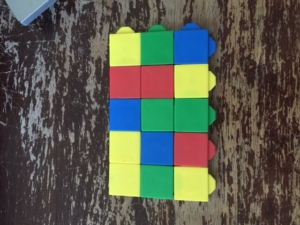A graph paper array.Quick picture of an array with the related equations.2. Arrays can be used to model both multiplication and division problems. They help relate to the two operations.

Here is an example of using a multiplication array to relate to division. Once the total is determined, students can see they can divide by the number of groups or rows, 2. Or they can divide by the number of columns or amount in each row, 3.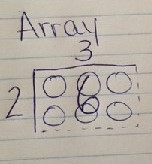3. It’s beneficial to use word problems that elicit organizing an object into equal rows or columns to encourage students to model an array.

Example Multiplication Problems:
• “Riley was planting in his tomato garden. He planted 3 rows of tomato plants. There were 5 plants in each row. How many tomato plants did Riley plant in his garden?”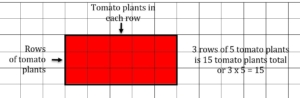• “Riley was planning in his tomato garden. He planted 3 tomato plants in each row. He planted 5 equal rows of tomatoes. How many tomato plants did Riley plant in his garden?”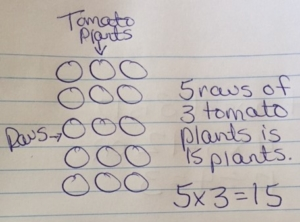Example Division Problems:
• “Rachel has 15 dolls. She has 5 shelves to place her dolls on. She wants an equal number of dolls on each shelve. How many dolls does Rachel place on each shelf?”• “Rachel has 15 dolls. She places 5 dolls on each row. How many rows does Rachel need to place all of her dolls on a shelf?”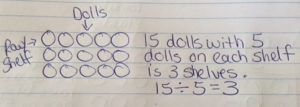4. Arrays help teach the commutative property of multiplication, which leads students to more efficient strategies for multiplying fluently.

This model shows 3 rows of 5, or 3 x 5.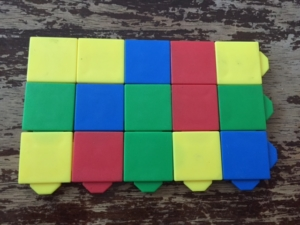This model shows 5 rows of 3, or 5 x 3Based on these two models, students can see the total or product stays the same because you are not adding or taking away any square tiles. The only thing that has changed is the way the rows and columns are organized, or the number of groups and how many in each group.

5. Array understanding in 3rd grade is essential for students developing understanding of area later in 3rd grade, and further in 4th grade. It also prepares students for modeling larger multiplication problems using partial products in 4th grade.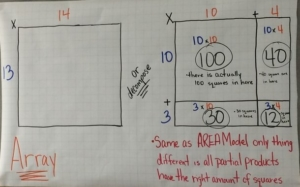A fun way to explore modeling arrays with multiplication and division problems is to give students bingo stampers and have them stamp the arrays on construction paper. You could pose the task, “There were 24 students that needed to be organized into equal rows for the assembly. How might their teacher have organized them into equal rows?” Then encourage students to record the division and multiplication equations for each array model.A great game to encourage students to practice modeling arrays is the “Array game.” Give partners a half sheet of graph paper, two different colors of crayons and a 0-9 number cube. Have the students take turns with their partner, rolling the number cube twice to determine the number of rows, and number of columns for their array. Then they will create their array, recording the equation that matches it and shading it their color. Play continues until a person can no longer create their array. Then the winner is whomever has the most square units shaded in their color crayon. (This can also be modified for division by creating division problem cards that students can pull, then create the array to match the given problem and solve for the quotient).

For more information on using arrays to model with multiplication and division problems or lessons, visit the websites:
http://investigations.terc.edu/library/curric-math/qa-1ed/teaching_mult_div.cfm
https://learnzillion.com/lesson_plans/5215-solve-division-problems-using-arrays
https://nrich.maths.org/8773
https://www.eduplace.com/math/mw/background/3/08/te_3_08_overview.html

For a lesson incorporating the use of arrays on CPalms, visit this website.